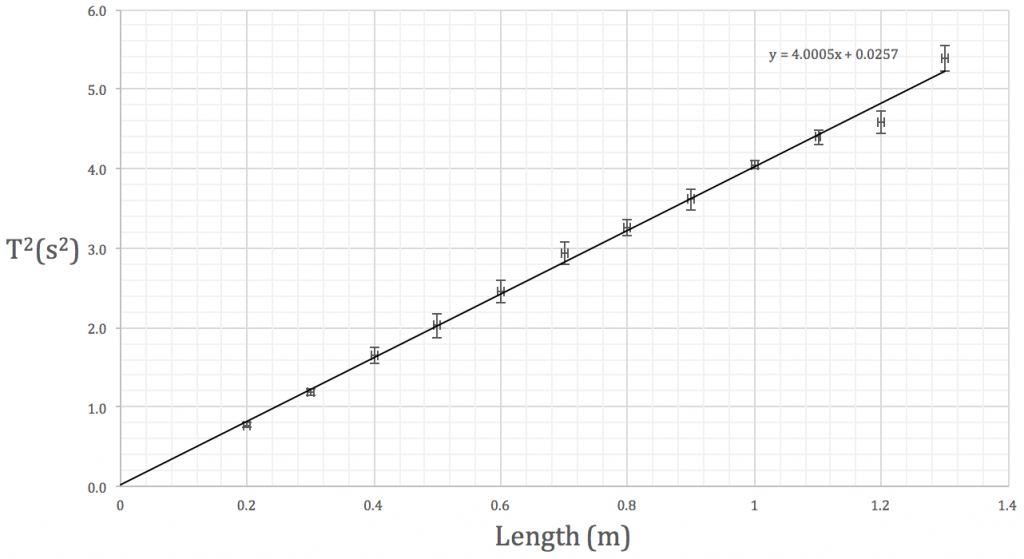using linest to obtain a gradient and uncertainty

The period T of a simple pendulum can be calculated using$T=2 \pi \displaystyle \sqrt{l \over g}$

where l is the pendulum length and g is the gravitational field strength.

Using a single value of length and period, we can determine the acceleration due to gravity.  However, it would be better experimental practise to vary the length of the pendulum and plot a graph of$T^2$ against length, determining g from the gradient of the line of best fit.You’re going to spend the next few periods analysing your simple pendulum data.  The attached pdf will walk you through the steps.  It would be better if you used your own results but I’ve put some sample data on the first page if you’ve forgotten to bring yours.

If you are using your chromebook, there may be subtle differences from the Excel instructions I have provided.  Let me know if anything doesn’t work and I’ll try to help.

Note that if you are using your own data, there will be no random uncertainty as measurements were not repeated.

Using Excel for your LO3 and Investigation

We’ll spend the next two lessons in the library learning how to use Excel.  Download the instructions using the link below.  If you have your own LO3 data, feel free to work with those values instead of the numbers I have provided.

By the end of this activity you will be able to;

• manipulate raw data using formulae in cells
• plot a graph of your results
• add a line of best fit

AH Physics – using excel

Here is the file you will need for the graphing activity in today’s lesson.

AH – help using Excel

This tutorial will help you with adding a line of best fit to your LO3 graph.  If you use the linest function, you can extract useful information on gradient and intercept of the line.  There are also screenshots to show how error bars can be added to individual points.

Excel Tutorial: Using LINEST function, Plotting a graph, Adding Error Bars